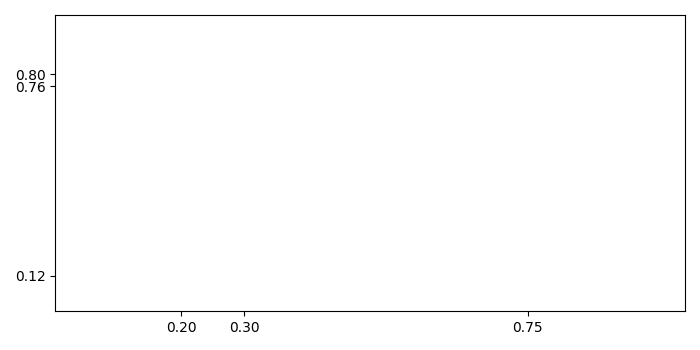# How to change the spacing between ticks in Matplotlib?

To set ticks on a fixed position or change the spacing between ticks in matplotlib, we can take the following steps −

• Create a figure and add a set of subplots.

• To set the ticks on a fixed position, create two lists with some values.

• Use set_yticks and set_xticks methods to set the ticks on the axes.

• To display the figure, use show() method.

## Example

import matplotlib.pyplot as plt
plt.rcParams["figure.figsize"] = [7.00, 3.50]
plt.rcParams["figure.autolayout"] = True
fig, ax = plt.subplots()
xtick_loc = [0.20, 0.75, 0.30]
ytick_loc = [0.12, 0.80, 0.76]
ax.set_xticks(xtick_loc)
ax.set_yticks(ytick_loc)
plt.show()

## Output